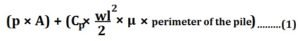# Formula To Find The Length Of A Pile

### Formula To Find The Length Of A Pile:

Let the properties of the soil penetrated be represented by the symbols given below:

Weight of the soil = w kg/cu.m

Angle of repose of soil = ϕ

Co-efficient of friction between concrete and soil = μ

Bearing capacity of the soil at the foot of the pile = p

If P be the load bearing capacity of the pile and h be its depth  of penetration, by Rankine’s theory we have

P = p × area of the cross section of pile + frictional resistance of pile

READ -  How To Calculate Shuttering Area For Construction

=Where A = area of cross-section of the pile and

Cp = coefficient of pressure, which isFor wet soil, Q may be assumed to vary between 15° to 22° and u between 0.3 to 0.35.

For known values of ϕ, μ, p,w, A and P, h can be determined by equation 1.